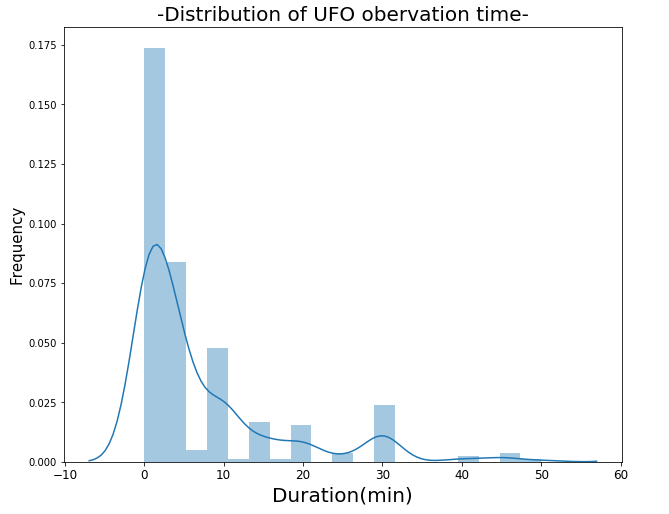﻿ Pandas Datetime: Create a plot of distribution of UFO observation time - w3resource

# Pandas Datetime: Create a plot of distribution of UFO observation time

## Pandas Datetime: Exercise-20 with Solution

Write a Pandas program to create a plot of distribution of UFO (unidentified flying object) observation time.

Sample Solution:

Python Code:

``````import pandas as pd
import matplotlib.pyplot as plt
import seaborn as sns
df['duration_sec'] = (df['length_of_encounter_seconds'].astype(float))/60
s = df["duration_sec"].quantile(0.95)
temp = df['duration_sec']
temp = temp.sort_values()
temp = temp[temp < s]
plt.figure(figsize=(10, 8))
sns.distplot(temp)
plt.xlabel('Duration(min)', fontsize=20)
plt.ylabel("Frequency", fontsize=15)
plt.xticks(fontsize=12)
plt.title("-Distribution of UFO obervation time-", fontsize=20)
plt.show()
``````

Sample Output:

```C:\Users\User\Anaconda3\lib\site-packages\scipy\stats\stats.py:1713: FutureWarning: Using a non-tuple sequence for multidimensional indexing is deprecated; use `arr[tuple(seq)]` instead of `arr[seq]`. In the future this will be interpreted as an array index, `arr[np.array(seq)]`, which will result either in an error or a different result.
return np.add.reduce(sorted[indexer] * weights, axis=axis) / sumval```

Python Code Editor:

Have another way to solve this solution? Contribute your code (and comments) through Disqus.

What is the difficulty level of this exercise?

﻿

## Python: Tips of the Day

F strings:

It is a common practice to add variables inside strings. F strings are by far the coolest way of doing it. To appreciate the f strings more, let's first perform the operation with the format function.

```name = 'Owen'
age = 25
print("{} is {} years old".format(name, age))
```

Output:

```Owen is 25 years old
```

We specify the variables that go inside the curly braces by using the format function at the end. F strings allow for specifying the variables inside the string.

```name = 'Owen'
age = 25
print(f"{name} is {age} years old")
```

Output:

```Owen is 25 years old
```

F strings are easier to follow and type. Moreover, they make the code more readable.

```A, B, C = {2, 4, 6}
print(A, B, C)
A, B, C = ['p', 'q', 'r']
print(A, B, C)
```

Output:

```2 4 6
p q r
```